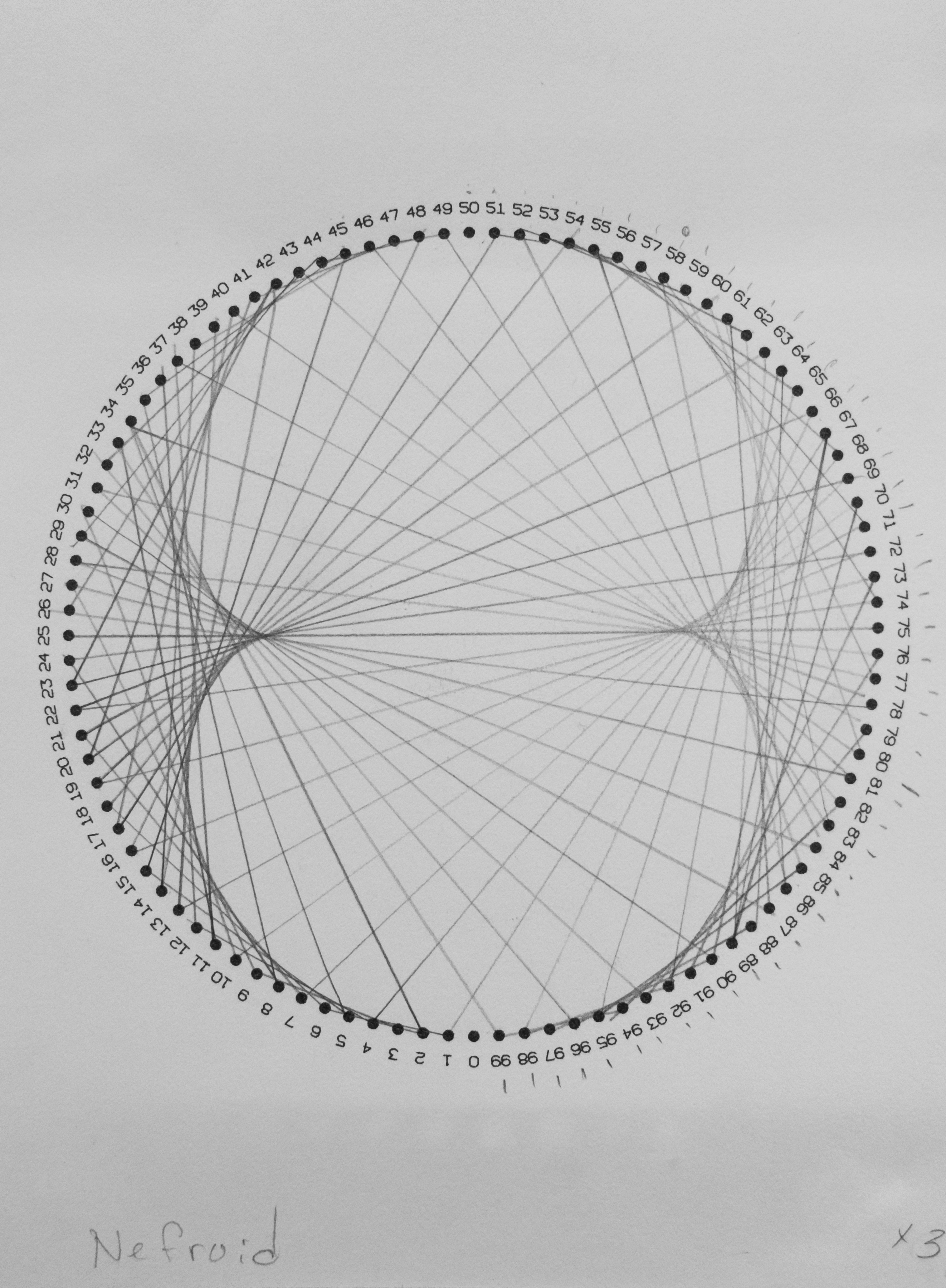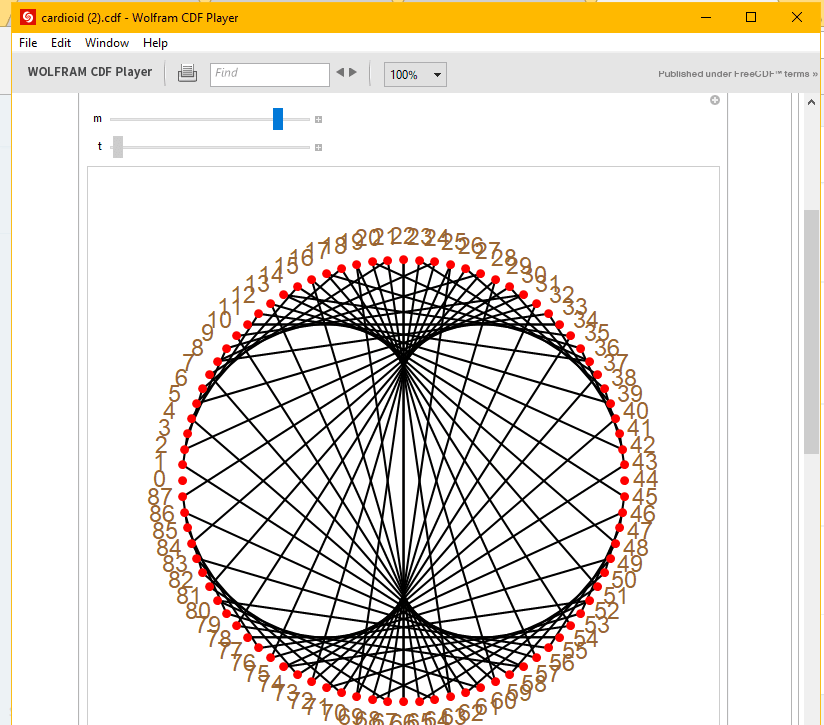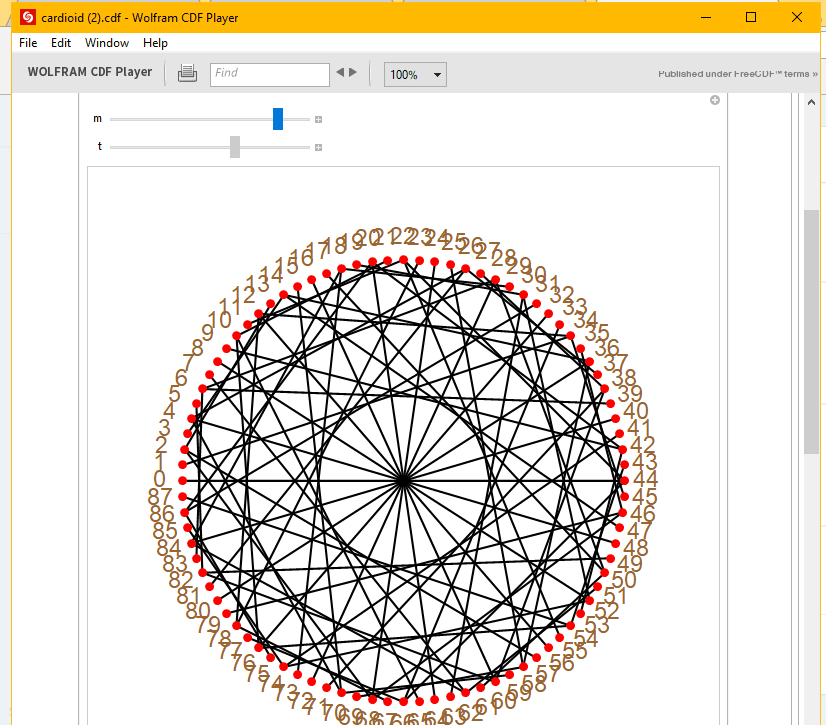# Multiplication Circles

Last year sometime the oldest and I did a short unit on multiplication circles. Here are some resources for that unit.

The first thing that I had him do was do the multiplication circle for the 2’s table and 3’s table. For the 2’s table, we connected the dots of the number we were multiplying and the product.  So for 1 that was 2 because 1 X 2 = 2. For 2 that was 4, because 2 x 2 = 4. For 3, 6 and 4, 8, and 5 10, and so forth. This takes a little patience.  One has to have the ability to keep up with where you are in the scope of 1 to 100 and to draw lines with a ruler easily.  Even my 12-year-old (at the time) was frustrated by this.  We then noticed what we saw, and predicted what the next sequence would look like.Here is the 100 dot circle that I used.

Afterwards I showed him this video by Mathologer.

I also found this awesome downloadable program that has a model allowing you to play and manipulate the multiplication circles.Here is the program.

Here is the model of the multiplication circles.1.Emily says:
1.The Beauty of Play says: